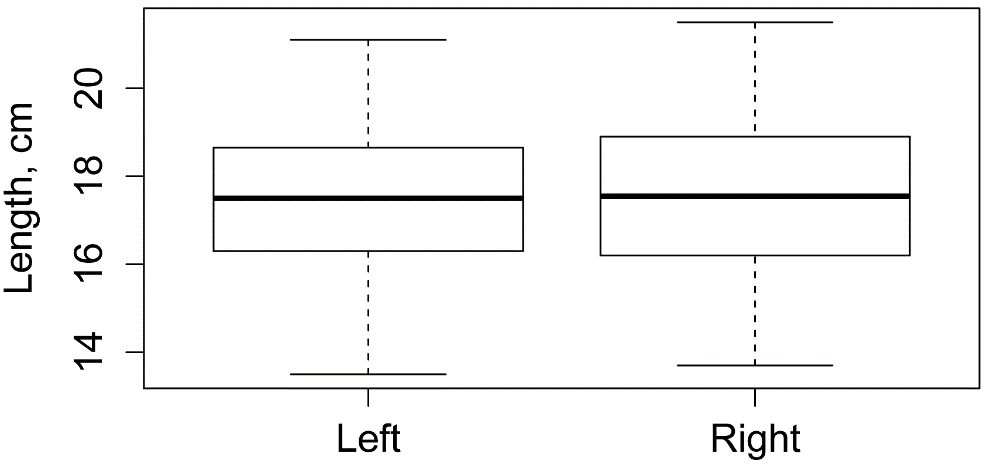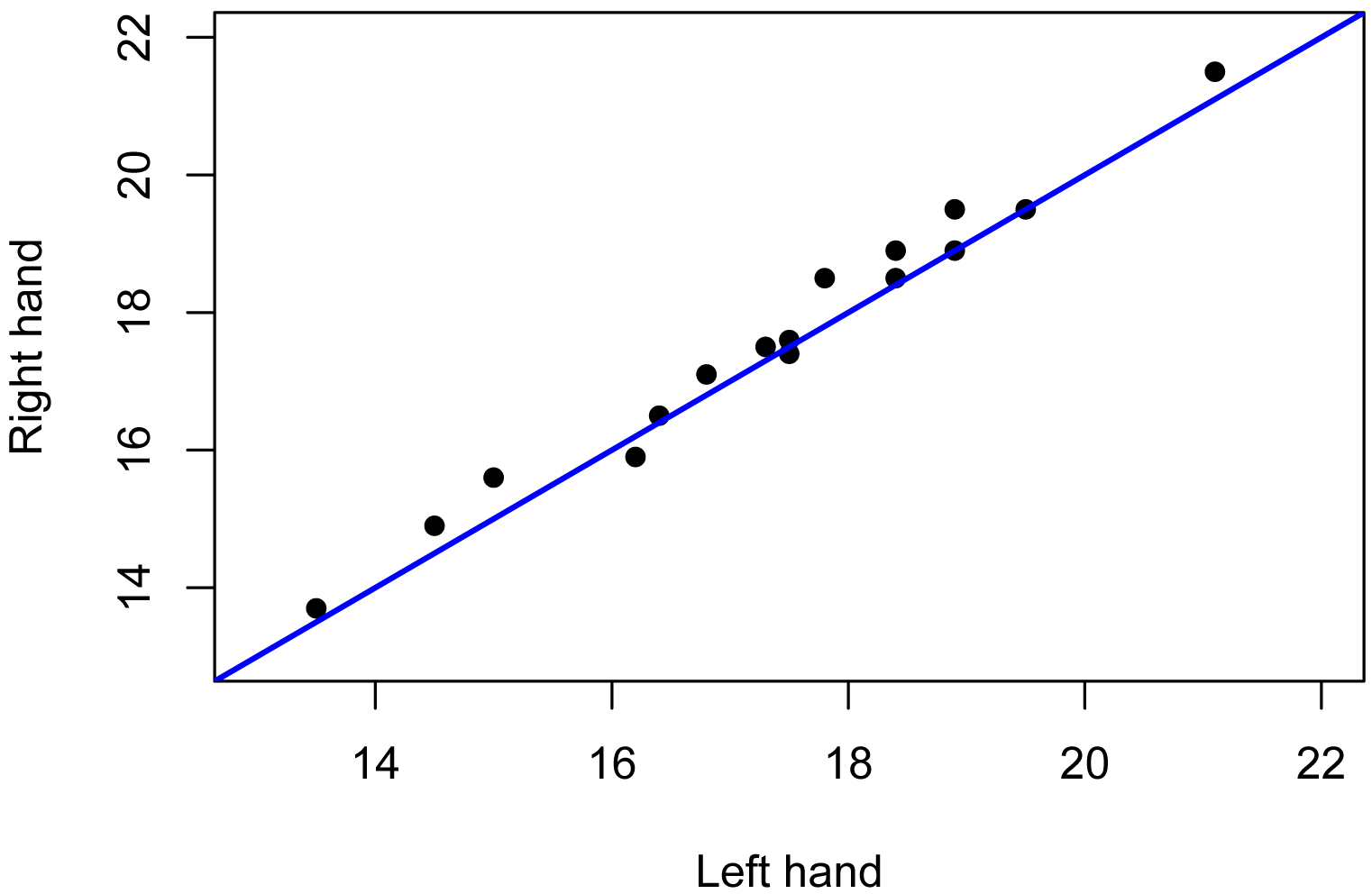## Summary and Analysis of Extension Program Evaluation in R

Salvatore S. Mangiafico

# Introduction to Permutation Tests

Permutation tests are increasingly common tests to perform certain types of statistical analyses.  They do not rely on assumptions about the distribution of the data, as some other tests do.  They are therefore considered to be nonparametric tests.  It is my understanding, however, that for certain tests—for example those testing a difference in means—that there are assumptions about the underlying data.

Permutation tests work by resampling the observed data many times in order to determine a p-value for the test.  Recall that the p-value is defined as the probability of getting data as extreme as the observed data when the null hypothesis is true. If the data are shuffled many times in accordance with the null hypothesis being true, the number of cases with data as extreme as the observed data could be counted, and a p-value calculated.

The advantages of permutation tests are

•  the lack of assumptions about the distribution of the underlying data,

•  their flexibility in the kinds of data they can handle (nominal, ordinal, interval/ratio),

•  and their being relatively straightforward to conduct and interpret.

The disadvantages of permutation tests are

•  the limited complexity of designs they can handle

•  the unfamiliarity with them for many readers.

#### The coin package

Permutation tests in this book will use the coin package, with either of two functions, independence_test and symmetry_test.  This book with use permutation tests with ordinal dependent variables, but the coin package is able to handle nominal, ordinal, and interval/ratio data.

A few notes on using permutation tests:

•  If the dependent variable is to be treated as an ordinal variable, it must be coded as an ordered factor variable in R.  It does not need to have numerals for levels.  For example it could have levels doctorate  >  masters  >  bachelors   >   associates  >  high.school.  But also it could have the levels 5 > 4 > 3 > 2 > 1

•  The general interpretation for significant results of these models isn’t that there is a difference among medians, but that there is a significant effect of the independent variable on the dependent variable, or that there is a significant difference among groups.

•  Post-hoc tests for factors or groups can be conducted with pairwise tests of groups.  The appropriate functions in the rcompanion package are pairwisePermutationTest, pairwisePermutationMatrix, pairwisePermutationSymmetry, and pairwisePermutationSymmetryMatrix.

•  Permutation tests for data arranged in contingency tables are presented in the Association Tests for Ordinal Tables chapter.

### Packages used in this chapter

The packages used in this chapter include:

•  coin

The following commands will install these packages if they are not already installed:

if(!require(coin)){install.packages("coin")}

### Permutation test example

The following example uses the left hand and right hand data from the Independent and Paired Values chapter.  For this example, we are interested in comparing the length of left hands and rights from 16 individuals.  First we will compare the left hands to right hands as independent samples (analogous to a Mann–Whitney test or t-test), then as paired values for each individual (analogous to a paired rank sum test or paired t-test).

Input = ("
Individual  Hand     Length
A           Left     17.5
B           Left     18.4
C           Left     16.2
D           Left     14.5
E           Left     13.5
F           Left     18.9
G           Left     19.5
H           Left     21.1
I           Left     17.8
J           Left     16.8
K           Left     18.4
L           Left     17.3
M           Left     18.9
N           Left     16.4
O           Left     17.5
P           Left     15.0
A           Right    17.6
B           Right    18.5
C           Right    15.9
D           Right    14.9
E           Right    13.7
F           Right    18.9
G           Right    19.5
H           Right    21.5
I           Right    18.5
J           Right    17.1
K           Right    18.9
L           Right    17.5
M           Right    19.5
N           Right    16.5
O           Right    17.4
P           Right    15.6
")

###  Check the data frame

Data

str(Data)

summary(Data)

### Remove unnecessary objects

rm(Input)

### Summarize data

library(FSA)

Summarize(Length ~ Hand,
data=Data,
digits=3)

Hand  n nvalid   mean    sd  min    Q1 median    Q3  max percZero
1  Left 16     16 17.356 1.948 13.5 16.35  17.50 18.52 21.1        0
2 Right 16     16 17.594 1.972 13.7 16.35  17.55 18.90 21.5        0

#### Box plot

boxplot(Length ~ Hand,
data=Data,
ylab="Length, cm")#### Scatter plot with one-to-one line

In the plot below, each point represents a pair of paired values.  Points that fall above and to the left of the blue line indicate cases for which the value for Right was greater than for Left.

Left  = Data\$Length[Data\$Hand=="Left"]

Right = Data\$Length[Data\$Hand=="Right"]

plot(Left, Right,
pch = 16,                # shape of points
cex = 1.0,               # size of points
xlim=c(13, 22),          # limits of x axis
ylim=c(13, 22),          # limits of y axis
xlab="Left hand",
ylab="Right hand")

abline(0,1, col="blue", lwd=2) # line with intercept of 0 and slope of 1#### Permutation test of independence

This test treats the two groups (left hand and right hand) as independent samples, and tests if there is a difference in values between the two groups.  The box plot above reflects the approach of this test.

library(coin)

independence_test(Length ~ Hand,
data = Data)

Asymptotic General Independence Test

Z = -0.34768, p-value = 0.7281

#### Permutation test of symmetry

This test treats the two groups (left hand and right hand) as having paired or repeated data, paired within Individual.  That is, the test looks at the difference between left hand and right hand for each individual.  The scatter plot above reflects the approach of this test.

Note the use of the symmetry_test function.

library(coin)

symmetry_test(Length ~ Hand | Individual,
data = Data)

Asymptotic General Symmetry Test

Z = -2.6348, p-value = 0.008418